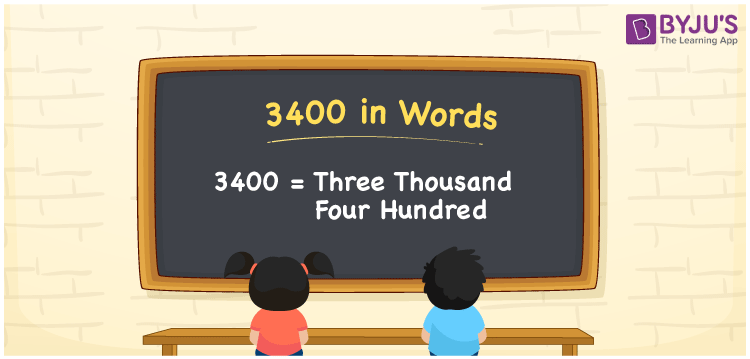# 3400 in Words

Three Thousand Four Hundred” is the word for the number 3400. The number 3400 in words is written as “Three thousand four hundred” in both the International and Indian Systems of Numerals. 3400 is a cardinal number since it expresses the value or quantity of objects. For example, if you made a transaction of Rs. 3400, you can write it as “I made a transaction of Rs. Three Thousand Four Hundred. Now, let us have a look at the spelling of 3400 in words with a complete explanation.

 3400 in Words: Three Thousand Four Hundred. Three Thousand Four Hundred in Numerical Form: 3400.

## 3400 in English Words

The number 3400 is spelt as “Three thousand four hundred” in English.## How to Write 3400 in Words?

We can write the number 3400 in words using the place value chart. Let’s make a place value chart to write the number 3400 in English.

 Thousands Hundreds Tens Ones 3 4 0 0

The expanded form of 3400 is as follows:

= 3 × Thousand + 4 × Hundred + 0 × Ten + 0 × One

= 3 × 1000 + 4 × 100

= 3000 + 400

= 3400

= Three thousand four hundred

Hence, 3400 in words is Three thousand four hundred.

The number 3400 is a natural number that lies between 3399 and 3401.

3400 in words – Three thousand four hundred

Is 3400 an odd number? – No

Is 3400 an even number? – Yes

Is 3400 a perfect square number? – No

Is 3400 a perfect cube number? – No

Is 3400 a prime number? – No

Is 3400 a composite number? – Yes

## Frequently Asked Questions on 3400 in Words

Q1

### How to write 3400 in words?

3400 in words is three thousand four hundred.

Q2

### Simplify 3000 + 400, and express it in words.

Simplifying 3000 + 400, we get 3400. Hence, 3400 in words is three thousand four hundred.

Q3

### Is 3400 a prime number?

No, 3400 is not a prime number.